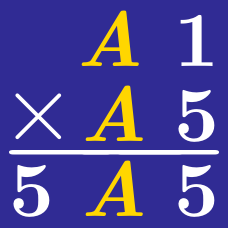Logic

# Cryptograms: Level 2 Challenges

$\Large \begin{array} {c c c c } & & \color{#69047E}{X}& \color{#69047E}{X} \\ & & \color{#D61F06}{Y} & \color{#D61F06}{Y} \\ + & & \color{#3D99F6}{Z} & \color{#3D99F6}{Z} \\ \hline & \color{#69047E}{X} & \color{#D61F06}{Y} & \color{#3D99F6}{Z} \\ \end{array}$

If each letter represents a distinct digit, what is the value of the three-digit number $\overline{XYZ}?$

$\begin{array}{ccccc} & & & & A&B\\ \times & & & & A &A \\ \hline & & B & A & A &B \end{array}$

Solve the above cryptogram. What is the first two-digit number in the product above, $\overline{AB}$?

Note: A number cannot start with 0, so A and B are non-zero.

$\begin{array} { l l l l l } & & & & & 9 & 9 & 9 \\ \times & & & & & A & B & C \\ \hline & & D & E & F & 1 & 3 & 2 \\ \end{array}$

In this cryptogram, $A,B,C,D,E$ and $F$ are (not necessarily distinct) single digits. What is the value of $A+B+C+D+E+F?$

$\begin{array} { l l l l l } & S & E & N & D \\ + & M & O & R & E \\ \hline M & O & N & E & Y \\ \end{array}$

In this cryptogram, each letter represents a distinct single digit positive integer except $O$ which is equal to 0. Find the value of $\overline{MONEY}.$

$\large{\begin{array}{cccccc} & & & A & B & C&D\\ \times & & & & & &D\\ \hline & & & D& C & B&A\\ \end{array}}$

Given that $A,B,C$ and $D$ are distinct single digit non-negative integers satisfying the cryptogram above, find $A+B+C+D$.

×